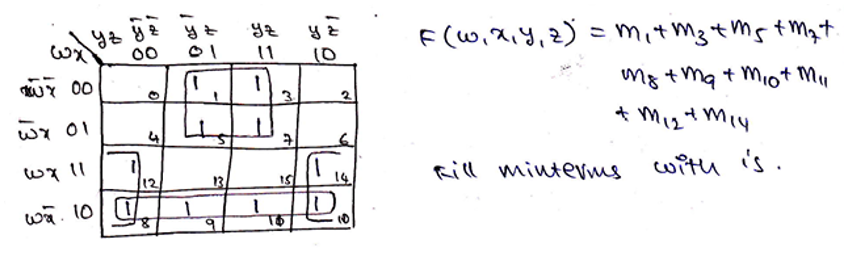# Mapping of expressions on K-Map and Encircling of groups

In this tutorial, we will learn about the mapping of expressions on K-Map and encircling of groups in Digital Electronics. By Saurabh Gupta Last updated : May 10, 2023

In the previous article (Karnaugh Map 2, 3 and 4- variable) we have already discussed the designing of K-Map and various forms in which they are represented based on either they are being mapped for minterm or maxterm.

In this article, we will be studying about, how do we map these minterm and maxterm expressions on the K-Map and how to encircle them into groups?

## Mapping of expressions on K-Map

1. In this article, the convention that will be followed is for mapping minterm expressions. Since minterm and maxterm expressions have a complementary relationship [ (POS of F) = (SOP of F')' ] we can also use minterm expressions to solve maxterm problems doing some manipulations.
2. During mapping of expressions on K-Map, one thing to be kept in the notice is that the upper LHS alphabet of K-Map always represents the MSB bits and the alphabets on the upper RHS denote LSB bits. For Ex: If we want to map m7 (7 is represented as 111 in Binary or in terms of minterms as ABC) on 3-variable K-Map, then A bit is the MSB bit and C is the LSB bit.
3. While mapping SOP expression, we put 1's in the blocks representing minterms in the K-Maps and 0's elsewhere.
4. While mapping POS expression, we put 0's in the blocks representing max terms in the K-Maps and 1's elsewhere.

## Encircling of Groups in a K-Map

After we map expressions in the K-Map, we encircle pairs, quads, and octets of 1's in the K-Map for the purpose of simplification of Boolean expressions.

### 1. Pairs

In 2, 3 and 4-variable K-Map, two adjacent 1's placed either vertically or horizontally are encircled. It is to be noted that diagonally adjacent 1's are never encircled.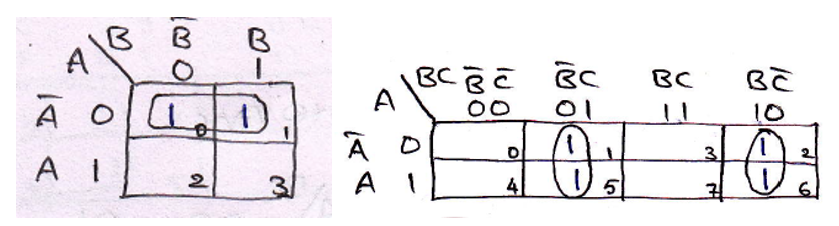In the K-Map, if four 1's are adjacent either vertically, horizontally or in the shape of a square, then these are encircled together.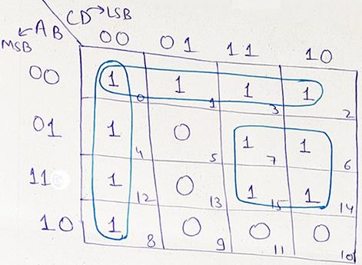### 3. Octets

The eight adjacent 1's are encircled in a K-Map are known as octets. They can be present either vertically or horizontally.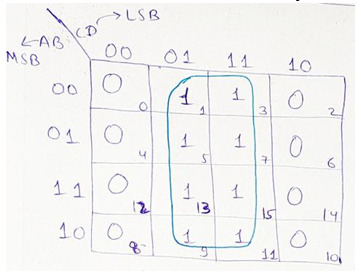### 4. Overlapping Groups

While circling the group of 1's, it is always kept in mind to encircle the group which has the largest number of 1's together, i.e., Octets are encircled first, then quads and pairs follow in the order. It is also possible that the same 1 may be used in more than one encircled group, these groups are known as overlapped groups.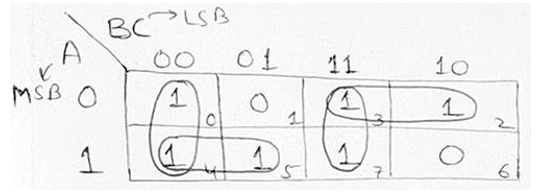### 5. Rolling Groups

While making a group of the largest number of 1's, it is possible to roll the K-Map. Rolling of groups can be made in such a way that LHS of the K-Map touches the RHS and top of the K-Map touches the bottom of the K-Map. Rolling is shown by half encircling the group on both sides. Rolling can be done to make obtain octet, quad or pair.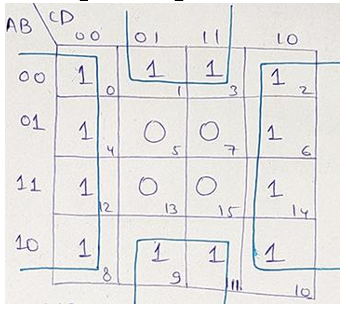### 6. Redundant Groups

While encircling the groups there may be a possibility that all the 1's of some group/groups are overlapped by other groups. Such a group is known as redundant groups, we need to eliminate these redundant groups. In the following given circle, the dotted encircled circle is the redundant circle, since all its elements are already covered in other groups.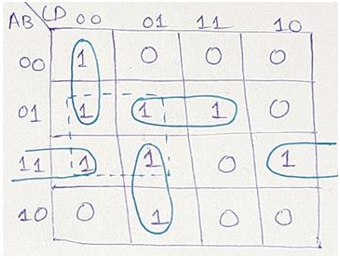## Examples

### Example 1

Map the Boolean function, F (A, B, C) = ∑ (1, 2, 5, 6) on three- variable K-Map and also encircle them into proper groups.

### Solution

From the above given Boolean function, we can infer that it is an expression for minterms. So, we will draw a K-Map used for SOP representation and place 1's where minterms are provided.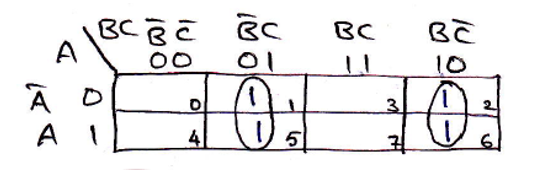### Example 2

Map the Boolean function, F (w, x, y, z) = ∑ (1, 3, 5, 7, 8, 9, 10, 11, 12, 14) on four variable K-Map and also encircle them into proper groups.

### Solution

From the above given Boolean function, we can infer that it is an expression for minterms. So, we will draw a K-Map used for SOP representation and place 1's where minterms are provided.